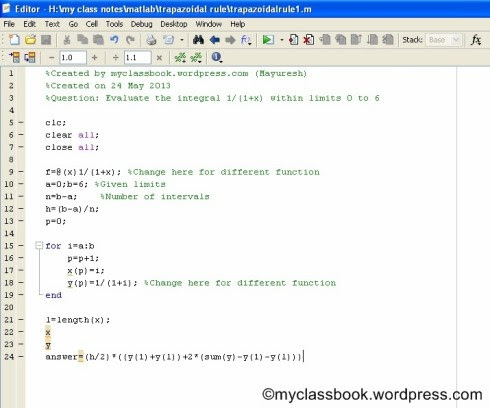# Trapezoidal Rule with MATLAB Program Example

## Trapezoidal Rule Derivation

The derivation for obtaining formula for Trapezoidal rule is given by,

## Example

Evaluate the integral x^4 within limits -3 to 3 using Trapezoidal rule.

## Solution

Let y(x)=x^4

here a=-3 and b=3

therefore (b-a)=6

let 'n' be the number of intervals. assume n=6 in this case.

also h=(b-a)/n = 6/6 =1

x: -3  -2  -1  0  1  2  3

y: 81  16  1  0  1  16  81

According to trapezoidal rule:

## MATLAB Program for Trapezoidal Rule

`%Created by myclassbook.org (Mayuresh) %Created on 24 May 2013 %Question: Evaluate the integral X^4 within limits 3 to -3clc; clear all; close all;f=@(x)x^4; %Change here for different function a=-3;b=3; %Given limits n=b-a; %Number of intervals h=(b-a)/n; p=0;for i=a:b  p=p+1;  x(p)=i;  y(p)=i^4; %Change here for different function endl=length(x); x y answer=(h/2)*((y(1)+y(l))+2*(sum(y)-y(1)-y(l)))`

## Example

Evaluate the integral 1/(1+x) within limits 0 to 6 using Trapezoidal rule.

## Solution

Let y(x)=1/(1+x)

here a=0 and b=6

therefore (b-a)=6

let 'n' be the number of intervals. assume n=6 in this case.

also h=(b-a)/n = 6/6 =1

x: 0                  1                    2              3                  4               5               6

y: 1.0000   0.5000   0.3333   0.2500   0.2000   0.1667   0.1429

According to trapazoidal rule:

### MATLAB code for the Trapazoidal rule:

`%Created by myclassbook.org (Mayuresh)%Created on 24 May 2013%Question: Evaluate the integral 1/(1+x) within limits 0 to 6clc; clear all; close all;f=@(x)1/(1+x); %Change here for different function a=0;b=6; %Given limits n=b-a; %Number of intervals h=(b-a)/n; p=0;for i=a:b  p=p+1;  x(p)=i;  y(p)=1/(1+i); %Change here for different function endl=length(x); x y answer=(h/2)*((y(1)+y(l))+2*(sum(y)-y(1)-y(l)))`

## Image Format

[caption id="" align="aligncenter" width="490"]MATLAB code for Trapezoidal Rule[/caption]

1.